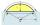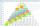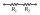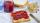# Expression of a variable from the formula + system of equations - math problems

#### Number of problems found: 101

• Simple equationsSolve system of equations: 5x+3y=5 5x+7y=25
• Non linear eqsSolve the system of non-linear equations: 3x2-3x-y=-2 -6x2-x-y=-7
• Height of the arc - formulaCalculate the height of the arc if the length of the arc is 77 and chord length 40. Does exist a formula to solve this?
• Together m+wWomen 30%. Men are 360 more. How many are together?
• Arithmetic progressionIn some AP applies: 5a2 + 7a5 = 90 s3 = 12 Find the first member a =? and difference d = ?
• GP - three membersThe second and third of a geometric progression are 24 and 12(c+1), respectively, given that the sum of the first three terms of progression is 76. determine the value of c.
• Geometric seqFind the third member of geometric progression if a1 + a2 = 36 and a1 + a3 = 90. Calculate its quotient.
• Two resistorsTwo resistors when they give 25 ohms in series and 4 ohms in parallel what the values of
• Hyperbola equationFind the hyperbola equation with the center of S [0; 0], passing through the points: A [5; 3] B [8; -10]
• Sequences AP + GPThe three numbers that make up the arithmetic sequence have the sum of 30. If we subtract from the first 5, from the second 4 and keep the third, we get the geometric sequence. Find AP and GP members.
• A bottleA bottle full of cola weighs 1,320 g. If we drink three-tenths of it, it will weigh 1,008g. How much does an empty bottle weigh?
• Rectangle from stringString 12m. Make rectangle when one side is two times longer than its width.
• Outside pointThe square ABCD and the point E lying outside the given square are given. What is the area of the square when the distance | AE | = 2, | DE | = 5 a | BE | = 4?
• FamilySon is 3/5 smaller than the mother. Mother is 99 cm higher than the son. How tall is the mother?
• JamJar with jam weighs 800 grams. Jam weighs three times as much as the empty glass. What is the weight of jam that is in the glass?
• In aIn a triangle, the aspect ratio a: c is 3: 2, and a: b is 5: 4. The perimeter of the triangle is 74cm. Calculate the lengths of the individual sides.
• Largest angle of the triangleCalculate the largest angle of the triangle whose sides have the sizes: 2a, 3/2a, 3a
• The rectangleThe rectangle has a circumference of 32 m. One side is 2 m longer than the other side. Calculate the side lengths of this rectangle.
• Four integersFnd four consecutive integers so that the product of the first two is 70 times smaller than the product of the next two.
• WineA bottle of wine costs 21 euros; and wine is 20 times more expensive than a bottle. How much a bottle cost?

Do you have an interesting mathematical word problem that you can't solve it? Submit a math problem, and we can try to solve it.

We will send a solution to your e-mail address. Solved examples are also published here. Please enter the e-mail correctly and check whether you don't have a full mailbox.

Please do not submit problems from current active competitions such as Mathematical Olympiad, correspondence seminars etc...

Do you have a system of equations and looking for calculator system of linear equations? Expression of a variable from the formula - math problems. System of equations - math problems.